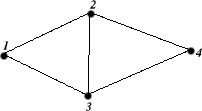# Reduction of GRAPH 3-COLORABILITY to Perl Regular Expression Matching

Credit: M-J. Dominus

## The GRAPH 3-COLORABILITY Problem

A graph G=(V, E) is 3-colorable if there is a mapping f from the vertices V to {red, green, blue} such that f(u) = f(v) only if u-v is not an element of E. The graph 3-colorability problem is: Given a graph, is it 3-colorable?

## Transformation to Regex Match

Let's represent the vertices of the graph as numbers between 1 and \$V. We also suppose that we have an adjacency list for the graph. The adjacency list contains [u, v] if the graph contains an edge u-v. So for example, the following graph:is represented as follows:

```	\$V = 4;
@E = ([1,2], [1,3], [2,3], [2,4], [3,4]);
```

Construct a string as follows:

```\$string = (join "\n", (("rgb") x \$V))
. "\n:\n"
. join "\n", (("rgbrbgr") x @E)
;
```

Now construct a regex as follows:

```\$regex =  '^'
.  (join "\\n", (".*(.).*") x \$V)
.  "\\n:\\n"
.  (join "\\n", map {".*\\\$_->\\\$_->.*"} @E)
.  '\$'
;
```

Now, the graph is 3-colorable if, and only if

```	\$string =~ /\$regex/;
```

is true. If so, the backreference variables \$1, \$2, etc., contain the color assignments for the corresponding vertices of the graph.

## Example

Suppose the graph is as depicted above. Then the value of \$string is as follows: (The newlines are significant.)

```	rgb
rgb
rgb
rgb
:
rgbrbgr
rgbrbgr
rgbrbgr
rgbrbgr
rgbrbgr
```

and the value of \$regex is:

```	^.*(.).*\n.*(.).*\n.*(.).*\n.*(.).*\n:\n.*\1\2.*\n.*\1\3.*\n.*\2\3.*\n.*\2\4.*\n.*\3\4.*\$
```

or, replacing the \n sequences with actual newlines for readability,

```	^.*(.).*
.*(.).*
.*(.).*
.*(.).*
:
.*\1\2.*
.*\1\3.*
.*\2\3.*
.*\2\4.*
.*\3\4.*\$
```

The test \$string =~ /\$regex/ succeeds, so the answer to the question is yes. Additionally, the match operator assigns the values b, g, r, and b to the special variables \$1, \$2, \$3, and \$4, indicating that a 3-coloring of the graph has vertices 1 and 4 colored blue, vertex 2 colored green, and vertex 3 colored red. For an example of a no result, add [1,4] to the edge list.

## Conclusion

Graph 3-colorability is NP-complete. If there were an efficient (polynomial-time) algorithm for computing whether or not a regex matched a certain string, we could use it to quickly compute solutions to the graph 3-colorability problem, and, by extension, to the knapsack problem, the travelling salesman problem, etc. etc.

## Reference

Michael R. Garey and David S. Johnson: Computers and Intractibility: A Guide to the Theory of NP-Completeness. 1979, W.H. Freeman and Company. ISBN 0-7167-1045-5. pp 84--87, 191.

mjd-perl-npc@plover.com